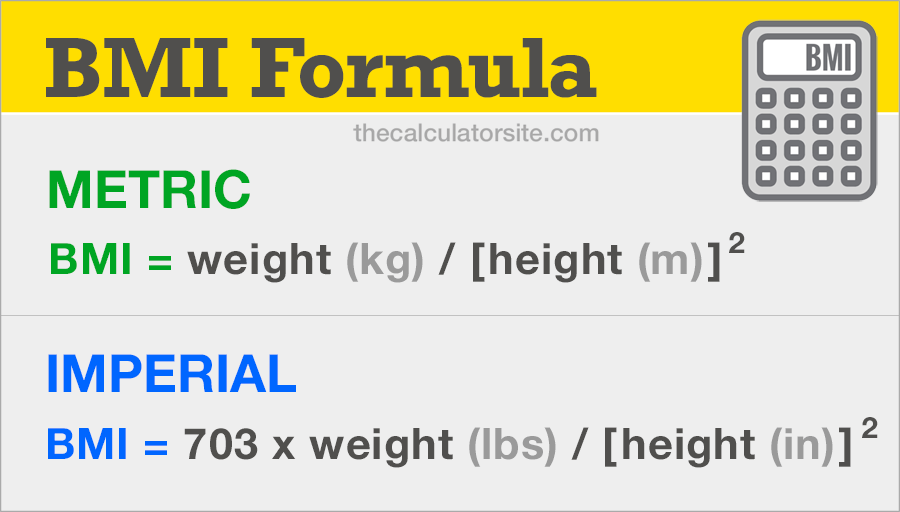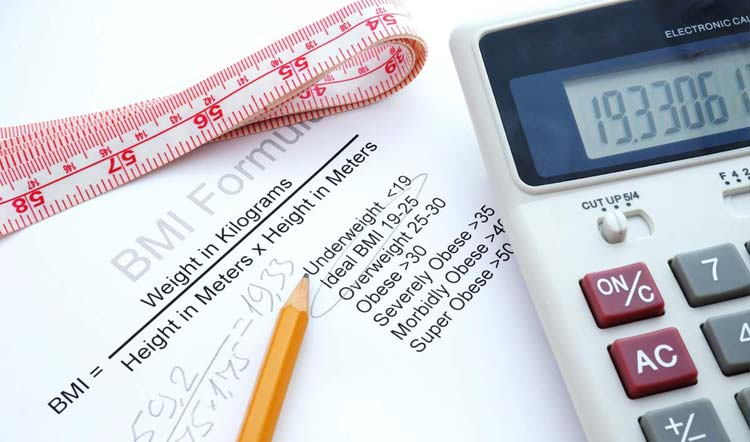# BMI Formula - How To Use The BMI Formula

| Last update: 06 October 2022

The BMI formula uses your weight (in kg or pounds) and your height (in meters or inches) to form a simple calculation that provides a measure of your body fat. The formula for BMI was devised in the 1830s by Belgian mathematician Adolphe Quetelet and is universally expressed in kg/m2.

Body mass index is a measure of body fat and is commonly used within the health industry to determine whether your weight is healthy. BMI applies to both adult men and women and is the calculation of body weight in relation to height. This article delves into the BMI formula and demonstrates how you can use it to calculate your own BMI.The first formula we've listed is the metric BMI formula, using kilograms and meters. The second one is the imperial BMI formula, which uses units of pounds and inches. Converters are available for kilos to stone, kilos to pounds and ounces and pounds, should you need them.

### Metric BMI Formula

BMI = weight (kg) / [height (m)]2

### Imperial BMI Formula

BMI = 703 × weight (lbs) / [height (in)]2

Let's go through a couple of examples to demonstrate how these formulae work.

## Using the BMI formula (metric units)

1. Multiply your height in meters (m) by itself
2. Divide your weight in kilograms (kg) by your step 1 result

### Example using formula

For an adult with height of 180 cm and weight of 75 kg. Our first step needs to be to convert the height into meters (British spelling: metres). As there are 100cm in a meter, we divide our figure by 100. This gives us 1.8m.

Let's plug those figures into our formula:

BMI = 75 ÷ (1.8 × 1.8)

BMI = 75 ÷ 3.24

This gives us a BMI figure of 23.15.

## Using the imperial BMI formula

Simple steps to work out your imperial BMI:

1. Multiply your height in inches (in) by itself
2. Divide your weight in pounds (lbs) by your step 1 result
3. Multiply the result from step 2 by 703.

### Example using formula

For an adult with height of 5ft 11 inches and weight of 155 pounds (lbs). Step one is to convert the height into inches only. There are 12 inches in a foot, so we simply multiply the 5ft by 12 and then add the 11 inches. This gives us a total of 71 inches.

Let's plug those figures into our formula:

BMI = 703 × (155 ÷ (71 × 71))

We do the multiplication inside the brackets first:

BMI = 703 × (155 ÷ 5041)

And then...

BMI = 703 × 0.030747867

This gives us a BMI figure of 21.62.

Of course, should you not wish to calculate BMI manually with the formula, you can use my popular BMI calculator or the interactive BMI formula below.

## Interactive BMI formula

Use the interactive BMI formula calculator below to demonstrate the formula and resulting BMI calculation for your chosen weight and height figures. Options for both metric and imperial units are available.

Please note that this calculator requires JavaScript to be enabled in your browser.

kg
cm

### Metric BMI Formula

BMI = weight (kg)
height (m)2
= A

Note that the results given by the Interactive BMI Formula should be used only as a guide and should not replace medical advice.

## BMI Categorization

The BMI statistical categories below are based on BMI scores and apply to adults of age 20 years and upwards. The World Health Organisation (WHO) regards a healthy adult BMI to be between 18.5 and 25.

BMI BMI Category
Less than 15 Very severely underweight
Between 15 and 16 Severely underweight
Between 16 and 18.5 Underweight
Between 18.5 and 25 Normal (healthy weight)
Between 25 and 30 Overweight
Between 30 and 35 Moderately obese
Between 35 and 40 Severely obese
Over 40 Very severely obese### BMI chart

For a full chart of BMI values, customised for your own height and weight, see my interactive BMI chart.

## The BMI formula remains controversial

We can't feature an article about the BMI formula without touching upon some of the controversies surrounding it.

It is a common argument that the results the BMI formula provides are too general and do not consider the gender, build, age or ethnicity of a person. For example, professional athletes are often considered overweight or obese when using BMI measurements due to their muscle content, which weighs more than fat.

Similarly, as people age their bone density decreases. So, although they may seem to have a weight within the normal BMI range, their measurement actually needs to be scaled-down to reflect this. In a study published in the Journal of Economics in 2008, John Cawley, professor at Cornell University, was able to demonstrate that, relative to percent body fat, BMI appears to misclassify substantial fractions of individuals as obese or non-obese. You can read more about his research in this HealthyDebate article published in 2016.

Alternatives to BMI do exist for those who consider BMI an inaccurate measurement tool.

It goes without saying that you should always speak to a Doctor or health professional for advice and guidance if you are concerned about your weight.